Wednesday, March 19, 2014

Fast and Accurate Implied Volatility Solver

The calibration of a stochastic volatility model or a volatility surface parameterization (like SVI) involves minimizing the model options volatilities against market options volatilities. Often, the model computes an option price, not an implied volatility. It is therefore useful to have a fast way to invert that option price to get back the implied volatility that corresponds to it. Furthermore during the calibration procedure, the model option price can vary widely: it is convenient to have a robust implied volatility solver.

Another more basic use of implied volatility solvers, is for the computation of Black-Scholes greeks for a given market option price.

A few years ago, P. Jaeckel produced a much better algorithm than a simple Newton or Brent solver, in his paper "By Implication". There is also a much simpler algorithm from Li, based on SOR and a good initial guess for SOR, which I found to be actually quite robust and fast. Now P. Jaeckel has a newer algorithm, faster and more accurate, close to double accuracy.

I have tested those on 1 million options of random volatility (between 0.001 and 3), random strikes (N deviations with a cap at 1M) for a few expiries.

In terms of performance, the results are independent of expiries, but in terms of accuracy, the new Jaeckel algorithm is particularly more accurate for the long-term options (5y and 15y).

Algorithm   Expiry  Vol RMSE  Price RMSE  Time
Jaeckel2014 5y      3.8E-16   2.1E-16     1.8s
Jaeckel2006 5y      1.3E-10   1.1E-10     3.0s
Jaeckel2014 15y     2.0E-12   2.4E-16     1.8s
Jaeckel2006 15y     1.5E-7    8.0E-11     2.7s

The maximum error from Jaeckel2006 is around 1e-8, while the one from Jaeckel2014 is 2e-15 (for very large unrealistic strike)

As a comparison, the simpler Li SOR-TS algorithm follows the given price accuracy independently of the expiry; I have tested with 1E-12. The error in implied volatility will be slightly higher: different close vols can give the same price due to the maximum achievable accuracy of the Black-Scholes formula with double numbers, even with a good cumulative normal distribution implementation. Its performance is however dependent on the number of deviations considered: closer to ATM means faster for Li algorithm.

Algorithm   Expiry  Deviation  Vol RMSE  Price RMSE  Time
Jaeckel2014 1y      5          4.2E-16   2.0E-17     1.8s
LiSORTS     1y      5          8.5E-9    1.9E-13     2.1s
Jaeckel2014 1y      3          3.1E-16   5.9E-17     1.7s
LiSORTS     1y      3          4.3E-12   1.6E-13     1.3s

Actually, I have cheated in my Li SOR-TS implementation: I have reused the idea from P. Jaeckel to compute the Black-Scholes price with erfc_x (unscaled erfc) instead of erfc. This simple change divides the number of exp calls by 2. Without this trick, for 5 deviations, SOR-TS took 3.6s (almost twice the time).

I would not be surprised if this was the main performance improvement between the two Jaeckels.

Fast and Accurate Implied Volatility Solver

The calibration of a stochastic volatility model or a volatility surface parameterization (like SVI) involves minimizing the model options volatilities against market options volatilities. Often, the model computes an option price, not an implied volatility. It is therefore useful to have a fast way to invert that option price to get back the implied volatility that corresponds to it. Furthermore during the calibration procedure, the model option price can vary widely: it is convenient to have a robust implied volatility solver.

Another more basic use of implied volatility solvers, is for the computation of Black-Scholes greeks for a given market option price.

A few years ago, P. Jaeckel produced a much better algorithm than a simple Newton or Brent solver, in his paper "By Implication". There is also a much simpler algorithm from Li, based on SOR and a good initial guess for SOR, which I found to be actually quite robust and fast. Now P. Jaeckel has a newer algorithm, faster and more accurate, close to double accuracy.

I have tested those on 1 million options of random volatility (between 0.001 and 3), random strikes (N deviations with a cap at 1M) for a few expiries.

In terms of performance, the results are independent of expiries, but in terms of accuracy, the new Jaeckel algorithm is particularly more accurate for the long-term options (5y and 15y).

Algorithm   Expiry  Vol RMSE  Price RMSE  Time
Jaeckel2014 5y      3.8E-16   2.1E-16     1.8s
Jaeckel2006 5y      1.3E-10   1.1E-10     3.0s
Jaeckel2014 15y     2.0E-12   2.4E-16     1.8s
Jaeckel2006 15y     1.5E-7    8.0E-11     2.7s

The maximum error from Jaeckel2006 is around 1e-8, while the one from Jaeckel2014 is 2e-15 (for very large unrealistic strike)

As a comparison, the simpler Li SOR-TS algorithm follows the given price accuracy independently of the expiry; I have tested with 1E-12. The error in implied volatility will be slightly higher: different close vols can give the same price due to the maximum achievable accuracy of the Black-Scholes formula with double numbers, even with a good cumulative normal distribution implementation. Its performance is however dependent on the number of deviations considered: closer to ATM means faster for Li algorithm.

Algorithm   Expiry  Deviation  Vol RMSE  Price RMSE  Time
Jaeckel2014 1y      5          4.2E-16   2.0E-17     1.8s
LiSORTS     1y      5          8.5E-9    1.9E-13     2.1s
Jaeckel2014 1y      3          3.1E-16   5.9E-17     1.7s
LiSORTS     1y      3          4.3E-12   1.6E-13     1.3s

Actually, I have cheated in my Li SOR-TS implementation: I have reused the idea from P. Jaeckel to compute the Black-Scholes price with erfc_x (unscaled erfc) instead of erfc. This simple change divides the number of exp calls by 2. Without this trick, for 5 deviations, SOR-TS took 3.6s (almost twice the time).

I would not be surprised if this was the main performance improvement between the two Jaeckels.

Monday, March 17, 2014

Arbitrage Free Interpolation of Option Prices using Piecewise Constant Density

Tension splines can produce in some cases arbitrage free C2 interpolation of options, but unfortunately this is not guaranteed. It turns out that, on some not so nice looking data, where the discrete probability density is not monotone but only positive, all previously considered interpolation fail (spline in volatility or variance, tension spline in log prices, harmonic spline on prices).

K     vol   put   b-slope  b-convexity
300.0 0.682 0.090 0.00e+00 0.00e+00
310.0 0.654 0.136 4.60e-03 0.00e+00
320.0 0.621 0.192 5.60e-03 1.00e-03
330.0 0.594 0.288 9.60e-03 4.00e-03
340.0 0.560 0.404 1.16e-02 2.00e-03
350.0 0.520 0.530 1.26e-02 1.00e-03
360.0 0.484 0.736 2.06e-02 8.00e-03
370.0 0.467 1.232 4.96e-02 2.90e-02
380.0 0.442 1.898 6.66e-02 1.70e-02
390.0 0.427 3.104 1.21e-01 5.40e-02
400.0 0.412 4.930 1.83e-01 6.20e-02

Possibly the simplest arbitrage free interpolation is to postulate the density as piecewise constant, ideally centered around each strike (if not centered, there is no guarantee that it will be positive). If a spline is used for interpolation, this means a quadratic spline. Unfortunately, because it is not C2, it then still fails to be arbitrage free.
It is also possible to price by integrating the payoff over the density. There is then one degree of freedom, the Fmin (minimum forward allowed before absorption) that can be adjusted so as to make the density always positive. This produces our only arbitrage free interpolation of the above input put option prices.
The implied volatility looks reasonable on this strange input: very much like a spline on volatilities. In contrast, the parabolic interpolator produces an oddly looking implied volatility shape, even though the density is in a way similar: piecewise constant. This is likely because I forced the second derivatives to match the discrete curvature, it is then not C1 in prices.

Unfortunately, the piecewise constant density interpolant can also produce some strange implied volatility shapes, for example on P. Jaeckel example data:
We find back the wiggles for large strikes. The lower end is particularly funny (which could be due to the fact that I don't have the data for low strikes). This is the corresponding density:

It appears then not so easy to produce a simple generally good looking interpolation.

Arbitrage Free Interpolation of Option Prices using Piecewise Constant Density

Tension splines can produce in some cases arbitrage free C2 interpolation of options, but unfortunately this is not guaranteed. It turns out that, on some not so nice looking data, where the discrete probability density is not monotone but only positive, all previously considered interpolation fail (spline in volatility or variance, tension spline in log prices, harmonic spline on prices).

K     vol   put   b-slope  b-convexity
300.0 0.682 0.090 0.00e+00 0.00e+00
310.0 0.654 0.136 4.60e-03 0.00e+00
320.0 0.621 0.192 5.60e-03 1.00e-03
330.0 0.594 0.288 9.60e-03 4.00e-03
340.0 0.560 0.404 1.16e-02 2.00e-03
350.0 0.520 0.530 1.26e-02 1.00e-03
360.0 0.484 0.736 2.06e-02 8.00e-03
370.0 0.467 1.232 4.96e-02 2.90e-02
380.0 0.442 1.898 6.66e-02 1.70e-02
390.0 0.427 3.104 1.21e-01 5.40e-02
400.0 0.412 4.930 1.83e-01 6.20e-02

Possibly the simplest arbitrage free interpolation is to postulate the density as piecewise constant, ideally centered around each strike (if not centered, there is no guarantee that it will be positive). If a spline is used for interpolation, this means a quadratic spline. Unfortunately, because it is not C2, it then still fails to be arbitrage free.
It is also possible to price by integrating the payoff over the density. There is then one degree of freedom, the Fmin (minimum forward allowed before absorption) that can be adjusted so as to make the density always positive. This produces our only arbitrage free interpolation of the above input put option prices.
The implied volatility looks reasonable on this strange input: very much like a spline on volatilities. In contrast, the parabolic interpolator produces an oddly looking implied volatility shape, even though the density is in a way similar: piecewise constant. This is likely because I forced the second derivatives to match the discrete curvature, it is then not C1 in prices.

Unfortunately, the piecewise constant density interpolant can also produce some strange implied volatility shapes, for example on P. Jaeckel example data:
We find back the wiggles for large strikes. The lower end is particularly funny (which could be due to the fact that I don't have the data for low strikes). This is the corresponding density:

It appears then not so easy to produce a simple generally good looking interpolation.

Tuesday, March 11, 2014

C2 Arbitrage Free Interpolation with Tension Splines

In a previous post, I have explored the arbitrage free wiggles in the volatility surface that P. Jaeckel found in his paper. I showed that interpolating in log prices instead of prices was enough to remove the wiggles, but then, it appears that the interpolation is not guaranteed to be arbitrage free, even though it often is. On another example from P. Jaeckel paper, that I reproduced inaccurately but well enough, it is not.

Using a Harmonic spline instead of a regular cubic spline seems to be enough to make the interpolation arbitrage free in practice (although not in theory as log-prices are not convex), but it is only C1.

One can use a tension spline instead. There is an algorithm from Pruess that updates the tension parameters so as to make the interpolation monotone and convex. It can be tweaked to ensure that the interpolation follows:
$$f'^2 + f'' \geq 0$$

This condition makes the interpolation on the log prices arbitrage free. As a first draft experiment, I just ensured numerically that the condition was valid, the resulting algorithm was fast enough and worked reasonably well.

Here is how the normalized density looks like with a simple harmonic spline on the call prices, a cubic spline in the logarithm of call prices, and an optimized tension spline in the logarithm of call prices.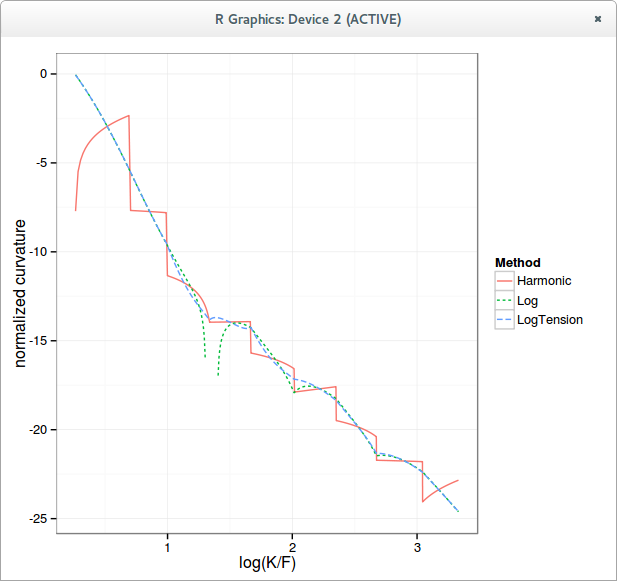logarithm of the curvature

The blank spot for the Log curve (spline) corresponds to negative curvature, that is, arbitrage. Notice how the tension spline is fully continuous, with no real spikes.
The implied volatility curve is very close to a more classic cubic spline interpolation on the implied variance.

Of course, the same kind of algorithm can be applied directly to the variance with a slightly different numerical test to ensure it is arbitrage-free, it might however be easier to find a more robust and more clever algorithm in the log prices.

C2 Arbitrage Free Interpolation with Tension Splines

In a previous post, I have explored the arbitrage free wiggles in the volatility surface that P. Jaeckel found in his paper. I showed that interpolating in log prices instead of prices was enough to remove the wiggles, but then, it appears that the interpolation is not guaranteed to be arbitrage free, even though it often is. On another example from P. Jaeckel paper, that I reproduced inaccurately but well enough, it is not.

Using a Harmonic spline instead of a regular cubic spline seems to be enough to make the interpolation arbitrage free in practice (although not in theory as log-prices are not convex), but it is only C1.

One can use a tension spline instead. There is an algorithm from Pruess that updates the tension parameters so as to make the interpolation monotone and convex. It can be tweaked to ensure that the interpolation follows:
$$f'^2 + f'' \geq 0$$

This condition makes the interpolation on the log prices arbitrage free. As a first draft experiment, I just ensured numerically that the condition was valid, the resulting algorithm was fast enough and worked reasonably well.

Here is how the normalized density looks like with a simple harmonic spline on the call prices, a cubic spline in the logarithm of call prices, and an optimized tension spline in the logarithm of call prices.logarithm of the curvature

The blank spot for the Log curve (spline) corresponds to negative curvature, that is, arbitrage. Notice how the tension spline is fully continuous, with no real spikes.
The implied volatility curve is very close to a more classic cubic spline interpolation on the implied variance.

Of course, the same kind of algorithm can be applied directly to the variance with a slightly different numerical test to ensure it is arbitrage-free, it might however be easier to find a more robust and more clever algorithm in the log prices.

Friday, March 07, 2014

Bachelier and Black-Scholes Fits of the Volatility Surface, what about SABR?

I always wondered if Bachelier was really worse than Black-Scholes in practice. As an experiment I fit various implied volatility surfaces with Bachelier and Black-Scholes and look at the average error in implied volatility by slice.

In theory Bachelier is appealing because slightly simpler: log returns are a bit more challenging to think about than returns. And it also takes indirectly into account the fact that OTM calls are less likely than OTM puts because of default risk, if you assume absorbing probability at strike 0.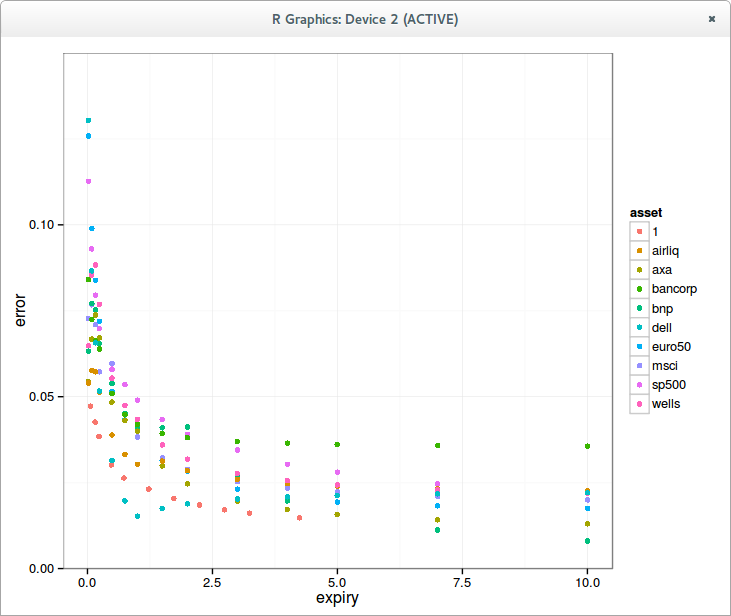BachelierBlack-Scholes
The error is in general smaller for Black-Scholes for short expiries, and higher for long expiries when compared to Bachelier. Interestingly, in theory, the difference of the models is more pronounced for longer expiries. One would have imagined that for very short expiries Bachelier would be equivalent to Black, it is not the case in term of fitting the smile.

Displaced diffusion (mixing both Bachelier and Black linearly) allows to gain x2 accuracy.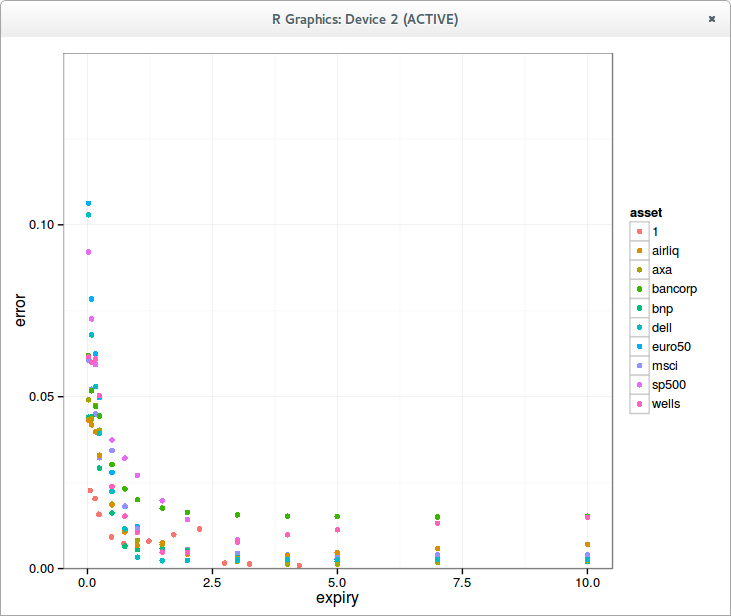Displaced Diffusion
What about SABR? Let's look at lognormal SABR, usually used for equities.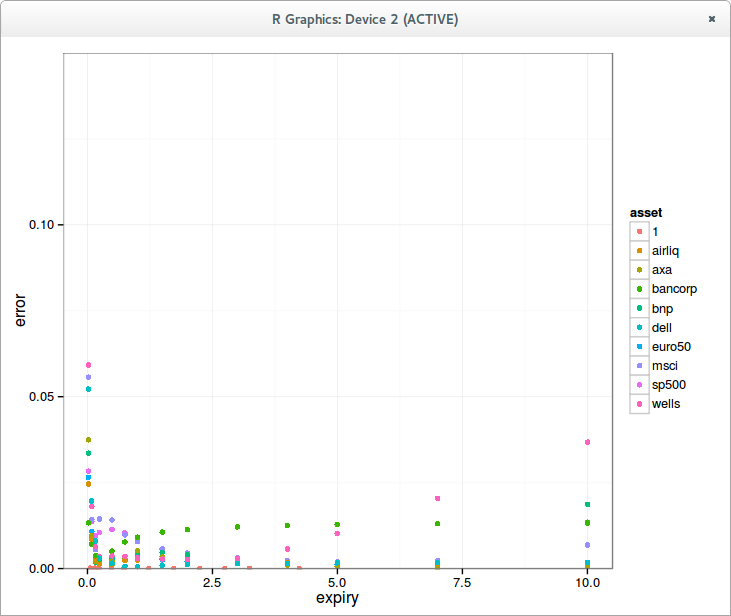Lognormal SABR (beta=1)
The fit is much better for long expiries, but not so great for a few outliers for long maturities, it can be actually worse than the simple displaced diffusion. A normal SABR might fix that.Normal SABR (beta=0) using Hagan lognormal formula
If one relies on the standard lognormal formula, the beta=0 SABR behaves very badly.Normal SABR using Hagan normal formula
This is fixed using the normal (bpvol) Hagan formula directly. The fit is then better overall for long maturities as expected from Black-Scholes vs Bachelier behavior.

If one look in log scale, the conclusion is not so obvious: beta=1 produces a better fit for a majority even for long expiries, but worse for a few (30% in my case) outliers.

What's clear however, is that one should never use the Black implied volatility Hagan formula with beta=0. This leaves a question on displaced SABR. Is displaced SABR is better suited than SABR with varying beta?

Bachelier and Black-Scholes Fits of the Volatility Surface, what about SABR?

I always wondered if Bachelier was really worse than Black-Scholes in practice. As an experiment I fit various implied volatility surfaces with Bachelier and Black-Scholes and look at the average error in implied volatility by slice.

In theory Bachelier is appealing because slightly simpler: log returns are a bit more challenging to think about than returns. And it also takes indirectly into account the fact that OTM calls are less likely than OTM puts because of default risk, if you assume absorbing probability at strike 0.BachelierBlack-Scholes
The error is in general smaller for Black-Scholes for short expiries, and higher for long expiries when compared to Bachelier. Interestingly, in theory, the difference of the models is more pronounced for longer expiries. One would have imagined that for very short expiries Bachelier would be equivalent to Black, it is not the case in term of fitting the smile.

Displaced diffusion (mixing both Bachelier and Black linearly) allows to gain x2 accuracy.Displaced Diffusion
What about SABR? Let's look at lognormal SABR, usually used for equities.Lognormal SABR (beta=1)
The fit is much better for long expiries, but not so great for a few outliers for long maturities, it can be actually worse than the simple displaced diffusion. A normal SABR might fix that.Normal SABR (beta=0) using Hagan lognormal formula
If one relies on the standard lognormal formula, the beta=0 SABR behaves very badly.Normal SABR using Hagan normal formula
This is fixed using the normal (bpvol) Hagan formula directly. The fit is then better overall for long maturities as expected from Black-Scholes vs Bachelier behavior.

If one look in log scale, the conclusion is not so obvious: beta=1 produces a better fit for a majority even for long expiries, but worse for a few (30% in my case) outliers.

What's clear however, is that one should never use the Black implied volatility Hagan formula with beta=0. This leaves a question on displaced SABR. Is displaced SABR is better suited than SABR with varying beta?

Monday, March 03, 2014

Arbitrage Free Wiggles

Peter Jaeckel, in a recent paper (pdf), shows that something that sounds like a reasonable arbitrage free interpolation can produce wiggles in the implied volatility slice.

The interpolation in question is using some convexity preserving spline on call and put option prices directly and in strike, assuming those input prices are arbitrage free. This is very similar to Kahale interpolation (pdf).

It seemed too crazy for me so I had to try out his example. And using a harmonic spline, it does produce arbitrage free wiggles.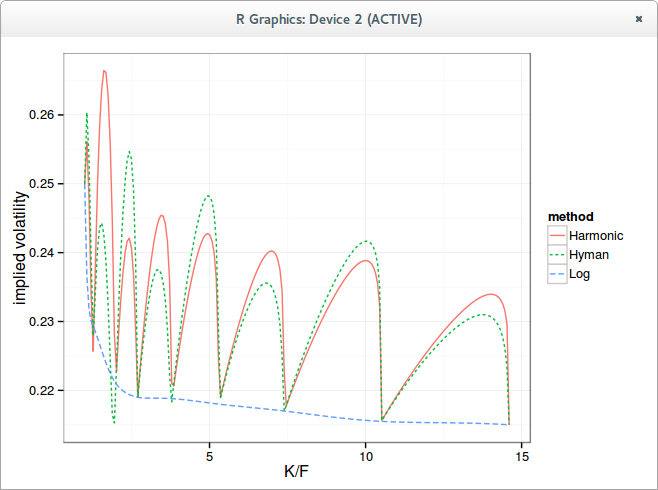Wiggles in the implied volatility
If we look at the probability density (the curvature), the Harmonic spline maintains a positive density, nearly piecewise flat in log scale, while Hyman, because it preserves only monotonicity, has some negative density which are cut out from the graph.Probability Density
In reality, if we look at the interpolation of prices in log scale, one can see that splines won't behave as expected at first on small numbers: they will give a much higher weight to the high values, producing something like a piecewise linear interpolation.

In reality, what one really wants for such data is to just interpolate the log prices with a spline, not the prices. This is the curve named "Log" in the graphs, where a simple cubic spline is used on the log prices, and fed to exp after interpolation.

Now it sounds like a reasonable arbitrage free interpolation would be to interpolate the discrete density log linearly, in a similar spirit as Hagan-West yield curve interpolation (pdf).

In general, if you interpolate very small numbers with a spline, you probably are doing something wrong.

Arbitrage Free Wiggles

Peter Jaeckel, in a recent paper (pdf), shows that something that sounds like a reasonable arbitrage free interpolation can produce wiggles in the implied volatility slice.

The interpolation in question is using some convexity preserving spline on call and put option prices directly and in strike, assuming those input prices are arbitrage free. This is very similar to Kahale interpolation (pdf).

It seemed too crazy for me so I had to try out his example. And using a harmonic spline, it does produce arbitrage free wiggles.Wiggles in the implied volatility
If we look at the probability density (the curvature), the Harmonic spline maintains a positive density, nearly piecewise flat in log scale, while Hyman, because it preserves only monotonicity, has some negative density which are cut out from the graph.Probability Density
In reality, if we look at the interpolation of prices in log scale, one can see that splines won't behave as expected at first on small numbers: they will give a much higher weight to the high values, producing something like a piecewise linear interpolation.

In reality, what one really wants for such data is to just interpolate the log prices with a spline, not the prices. This is the curve named "Log" in the graphs, where a simple cubic spline is used on the log prices, and fed to exp after interpolation.

Now it sounds like a reasonable arbitrage free interpolation would be to interpolate the discrete density log linearly, in a similar spirit as Hagan-West yield curve interpolation (pdf).

In general, if you interpolate very small numbers with a spline, you probably are doing something wrong.